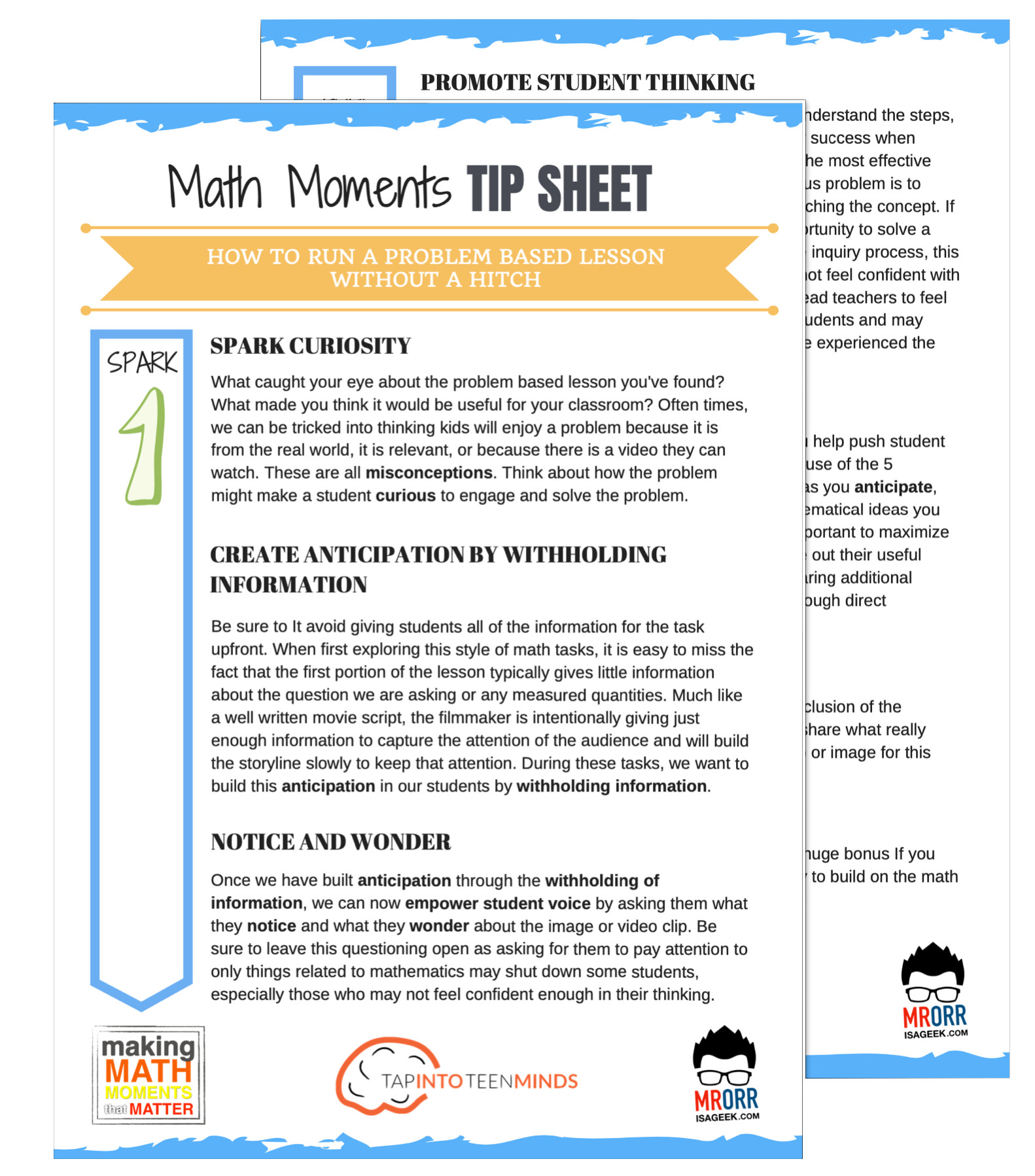Teach with Confidence# Make Math Moments Problem-Based Tasks & Units

• All
• Algebra
• Area
• Area of rectangles
• Area of squares
• Circles
• Circumference
• Classifying triangles
• Counting
• Data Literacy
• Data Management
• Decimals
• Division
• Equalities
• Estimation
• Fraction Multiplication
• Fractions
• Geometry
• Integers
• Linear Relations
• Linear Relationships
• Mean
• Measurement
• Multiplication
• Number
• Operations
• Order of Operations
• Partitive Division
• Patterning
• Percent
• Percentages
• Perimeter of triangles
• Probability
• Proportional Reasoning
• Pythagorean Theorem
• Rate
• Rate Reasoning
• Ratio Reasoning
• Ratios and Rates
• Rectangular Prisms
• Solving Equations
• Spatial Reasoning
• Subtraction
• Surface Area
• Systems of Equations
• Volume